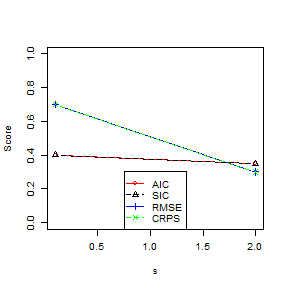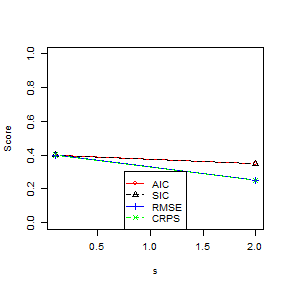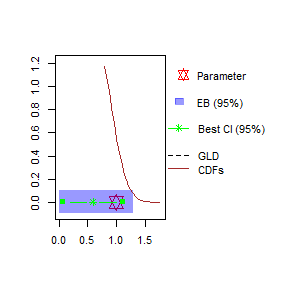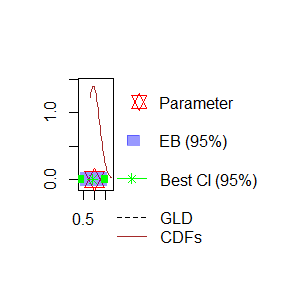# SUR: A simulation

library(ldt)
library(kableExtra)
library(MASS)

It is recommended to read the following vignette first:

## Introduction

In this vignette, we draw a sample from a known population and talk about the automatic variable selection in ldt (In another vignette we use an actual data set with a similar goal). In this vignette, we focus on a simulation problem with two endogenous variables and the following DGP: \begin{align} \label{eq:sur-sim-model} \begin{pmatrix}y_1\\y_2\end{pmatrix}=& \begin{bmatrix} 1 & 2 & 0\\ 0 & 3 & 4 \end{bmatrix}\begin{pmatrix}x_1\\x_2\\x_3\end{pmatrix} + \begin{pmatrix}e_1\\e_2\end{pmatrix}\\ &\begin{pmatrix}e_1\\e_2\end{pmatrix}=s \begin{bmatrix} 2 & 1\\ 0 & 2 \end{bmatrix}\begin{pmatrix}\epsilon_1\\\epsilon_2\end{pmatrix},\quad \epsilon_i\sim N(0,1),\\ &x_i\sim N(\frac{i}{3},1), \end{align}

where $$y_1$$ and $$y_2$$ are endogenous variables, $$x_i$$ for $$i=1,\dots,3$$ are exogenous variables, $$s$$ is a constant parameter, $$\epsilon_1$$ and $$\epsilon_2$$ are independent, and $$e_1$$ and $$e_2$$ are disturbances. It is easy to see that $$\operatorname{var}(e_1)=5s^2$$, $$\operatorname{var}(e_2)=4s^2$$, and $$\operatorname{cov}(e_1,e_2)=2s^2$$. Also, note that the model is restricted and each dependent variable is explained by 2 variables.

Furthermore, we assume that there is a sample of size $$n$$ for $$y_1$$ and $$y_2$$ and 18 other variables denoted by $$z_i$$, where, $\begin{equation} z_i\sim N(\frac{i}{18},1),\quad \text{ for } i=1,\dots,18. \end{equation}$ We assume that $$\{z_i\}$$ is the set of the potential regressors. Note that $$z_{6}$$, $$z_{12}$$ and $$z_{18}$$ are respectively equal in distribution to $$x_1$$, $$x_2$$ and $$x_3$$ (the means are $$1/3$$, $$0.5$$ and $$1$$, respectively).

In this example, we focus on the ability to select the true random variables and to estimate the parameters properly, in some scenarios on the $$s$$ and $$n$$:

sampleSizes <- c(30, 50)
sMultipliers <- c(0.1, 2)

Note that a larger value for $$s$$ (element of sMultipliers) means more dispersed and more correlated disturbances and a larger value for $$n$$ (element of sampleSizes) means more degrees of freedom.

We assume that the number of endogenous variables is known. Furthermore, to decrease the size of the model set, we assume that we know that the number of regressors is not larger than 3. In other words, we will use the following data (If you are not familiar with the arguments of the SurSearch_s() function, please read this vignette first):

xSizes <- list(as.integer(c(1, 2)), as.integer(c(3)))
xCounts <- c(NA, 5)
yGroups <- list(as.integer(c(1, 2)))

## Data

The following function can draw a sample from the described population:

GetSample <- function(n, s) {
z <- NULL
for (i in (1:18)) {
z <- cbind(z, rnorm(n, i / 18, 1))
}
z <- as.matrix(z)
x <- z[, c(6, 12, 18)]
e <- as.matrix(mvrnorm(n, c(0, 0), s * s * matrix(c(5, 2, 2, 4), 2, 2)))
y <- x %*% t(matrix(c(1, 2, 0, 0, 3, 4), 2, 3, byrow = TRUE)) + e
colnames(y) <- c("y1", "y2")
colnames(z) <- paste0("z", seq(1, 18, 1))
return(list(y = y, z = z))
}

Note that with x = z[,c(6,12,18)] we are assuming equality of random variables (not just equality in distribution).

## Variable selection

In each simulation, a sample is drawn randomly. Therefore, we need a seed for the random number generator:

seed <- 340
set.seed(seed)

Given the sample, we use SurSearch_s() function to find the best model. This function will select one best mode for each of the evaluation methods. We use the results for AIC, SIC, RMSE, and CRPS measures:

measureOptions <- GetMeasureOptions(
typesIn = c("aic", "sic"),
typesOut = c("rmse", "crps")
)
measureNames <- c("AIC", "SIC", "RMSE", "CRPS")

If you are not familiar with the arguments of the SurSearch_s() function, please read this vignette first. The measureNames vector is just a display name that we will use later.

In order to compare the performance of different measures, we use the following scoring schema:

GetScore <- function(indices1, indices2) {
if (length(indices1) == 2 && length(indices2) == 2 &&
all(indices1 == c(6, 12)) && all(indices2 == c(12, 18))) {
return(1)
}
score <- 0
if (any(indices1 == 6)) score <- score + 0.1
if (any(indices1 == 12)) score <- score + 0.1
if (any(indices2 == 12)) score <- score + 0.1
if (any(indices2 == 18)) score <- score + 0.1
return(score)
}

This schema uses the indices of the regressors in the (restricted) best model and calculates the score. If the indices belong to the true model (i.e., indices1=c(6,12) and indices2=c(12,18)), the score is 1. otherwise each correct index (i.e., 6 and 12 for the first equation and 12 and 18 for the second equation) adds 0.1 points to the score. For example, this means the maximum score for an incorrect model is 0.4. Such a model has all the true explanatory variables and some irrelevant ones. The minimum score is zero. This is the score of a model with no correct explanatory variable.

The SurSearch_s() function also reports the inclusion weights. The previous function is used to get the score of such a list. In this case, indices1 and indices2 are the index of the first 2 explanatory variables in the inclusion list.

Before we continue, we need a helper function to get the required indices from the estimations:

helper_GetIndices <- function(measureRes, measureSumRes) {
isRest1 <- measureSumRes$target1$model$bests$best1$coefs$isRestricted
isRest2 <- measureSumRes$target2$model$bests$best1$coefs$isRestricted

if (is.null(isRest1)) {
mindices1 <- sort(measureRes$target1$model$bests$best1$exoIndices) } else { mindices1 <- sort(measureRes$target1$model$bests$best1$exoIndices[isRest1[, 1] != 1])
}
if (is.null(isRest2)) {
mindices2 <- sort(measureRes$target2$model$bests$best1$exoIndices) } else { mindices2 <- sort(measureRes$target2$model$bests$best1$exoIndices[isRest2[, 2] != 1])
}

end <- nrow(measureRes$target1$model$inclusion) iindices1 <- sort(measureRes$target1$model$inclusion[3:end, 1, drop = F],
index.return = TRUE, decreasing = TRUE
)
iindices2 <- sort(measureRes$target2$model$inclusion[3:end, 1, drop = F], index.return = TRUE, decreasing = TRUE ) return(list( model1 = sort(mindices1), model2 = sort(mindices2), inclusion1 = sort(iindices1$ix[1:2]), inclusion2 = sort(iindices2$ix[1:2]) )) } The central piece of this experiment is the following function in which we generate a sample, search for the best models for each performance measure, and calculate the score of the best models: SampleAndEstimate <- function(i, res, n, s, xSizes, xCounts, yGroups, measureOptions, searchItems, checkItems) { sample <- GetSample(n, s) search_res <- SurSearch_s( y = sample$y, x = sample$z, numTargets = 2, xSizes = xSizes, counts = xCounts, yGroups = yGroups, searchSigMaxIter = 1, searchSigMaxProb = 0.1, searchItems = searchItems, measureOptions = measureOptions, modelCheckItems = checkItems, searchOptions = GetSearchOptions(printMsg = FALSE), savePre = NULL ) result_sum <- summary(search_res, y = sample$y, x = sample$z, test = FALSE, printMsg = FALSE) if (i < 0) { return(list(search_res = search_res, result_sum = result_sum)) } j <- 0 for (mea in c("aic", "sic", "rmse", "crps")) { j <- j + 1 h <- helper_GetIndices(search_res[[mea]], result_sum[[mea]]) res$model[[i, j]] <- res$model[[i, j]] + GetScore(h$model1, h$model2) res$inclusion[[i, j]] <- res$inclusion[[i, j]] + GetScore(h$inclusion1, h$inclusion2) } return(res) } Finally, we run the simulation: simCount <- 2 sur_sim_res <- list() for (n in sampleSizes) { res <- list(model = matrix(0, length(sMultipliers), length(measureNames), dimnames = list(paste0("sigma=", sMultipliers), measureNames) )) res$inclusion <- res$model i <- 0 for (s in sMultipliers) { i <- i + 1 for (iter in seq(1, simCount)) { seedi <- seed + iter measureOptions$seed <- seedi
measureOptions$simFixSize <- 6 measureOptions$trainRatio <- 0.75
searchItems <- GetSearchItems(model = TRUE, type1 = FALSE, bestK = 10, inclusion = TRUE)
res <- SampleAndEstimate(
i, res, n, s, xSizes, xCounts, yGroups, measureOptions,
searchItems, NULL
)
}
}
res$model <- res$model / simCount
res$inclusion <- res$inclusion / simCount
sur_sim_res[[length(sur_sim_res) + 1]] <- list(n = n, sMultipliers = sMultipliers, res = res)
} 

You can increase the number of simulations (We choose a small number to decrease the computation time). Note that for each n$$\in$$sampleSizes and each s$$\in$$sMultipliers, we sample and estimate 2 times. This is relatively time-consuming.

### Result

Comparing the performance of different measures in selecting the true explanatory variables can be interesting. Therefore, in the following plot we draw the scores of the best models:Scores in finding the true model (n=30, 50)

There is a similar result for the inclusion weights:Scores in finding the true inclusion variables (n=30, 100, 100)

## Estimation

Apart from finding the correct variables, another important task is estimating the unknown parameters. ldt provides 4 types of information about a parameter: - estimated coefficient and its estimated variance; - the value of the cumulative distribution function at some specific points; - extreme bounds; and - first four moments of the combined distribution. We specify these options by the following code:

searchItems <- GetSearchItems(
model = FALSE, type1 = TRUE,
bestK = 1, cdfs = seq(0.8, 1.8, 0.03),
extremeMultiplier = 2, mixture4 = TRUE
)

In SurSearch, type1 information is the estimated parameters. Note that in order to set a proper value for cdfs, we need to run the code (at least) twice. This is because we need to get some general idea about the range of values of estimated coefficients (e.g., by studying the extreme bounds analysis result).

Next, we select the values of $$n$$ and $$s$$:

s <- 1
n <- 50

Next, we want to estimate two types of coefficients: an unrestricted search and a restricted one in which we discard a part of the model set with low. We assess the performance by using AIC:

checkItems <- GetModelCheckItems(maxAic = 10)

Note that we adjusted the value of maxAic by running the code multiple times. This is because, at the first stage, we have no information about the magnitude of the AICs.


sur_sim_one <- list(
unr = SampleAndEstimate(
-1, NULL, n, s, as.integer(c(3)), c(NA), list(as.integer(c(1, 2))), measureOptions,
searchItems, checkItems
),
res = SampleAndEstimate(
-1, NULL, n, s, as.integer(c(3)), c(NA), list(as.integer(c(1, 2))), measureOptions,
searchItems, checkItems
)
) 

The number of estimated regressions for the unrestricted and restricted model sets are 136 and 18, respectively.

In this example, we present the results for one of the estimated parameters: $$\frac{\rho y_1}{\rho x_1}$$ in the following figure. Note that the actual value of this parameter is 1.0 (see the equation in the first section). Also, note that we use ldt::PlotCoefs() and ldt::GetGldFromMoments() functions.Estimated coefficient (searches with and without restricting AIC)